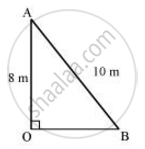# A ladder 10 m long reaches a window 8 m above the ground. Find the distance of the foot of the ladder from base of the wall. - Mathematics

A ladder 10 m long reaches a window 8 m above the ground. Find the distance of the foot of the ladder from base of the wall.

#### SolutionLet OA be the wall and AB be the ladder.

Therefore, by Pythagoras theorem,

AB2 = OA + BO

(10 m)2 = (8m)2 + OB2

100 m2 = 64 m2 + OB2

OB2 = 36 m2

OB = 6 m

Therefore the distance of the foot of the ladder from the base of the wall is 6 m

Concept: Right-angled Triangles and Pythagoras Property
Is there an error in this question or solution?

#### APPEARS IN

NCERT Class 10 Maths
Chapter 6 Triangles
Exercise 6.5 | Q 9 | Page 151